• matlab作概率密度函数很简单，但是本人学的python，因此想用python作图，从txt文本中读取数据，然后用python概率密度函数图像，
• 今天小编就为大家分享一篇python高斯分布概率密度函数的使用详解，具有很好的参考价值，希望对大家有所帮助。一起跟随小编过来看看吧
• 今天小编就为大家分享一篇python实现beta分布概率密度函数的方法，具有很好的参考价值，希望对大家有所帮助。一起跟随小编过来看看吧
• 概率密度函数 分布概率密度函数（PDF）。 随机变量的（PDF）为 其中alpha是形状参数， beta是速率参数。 安装 $npm install distributions-gamma-pdf 要在浏览器中使用，请使用 。 用法 var pdf = require ( '...JavaScript • 我让Python返回10000个参数为n和p的二项式随机变量 进行10000次实验，每次抛10次硬币，统计有几次正面朝上，最后统计每次实验正面朝上的次数 ''' binom_sim = stats.binom.rvs(n=10,p=0.3,size=10000) print(len... # coding: utf-8 import numpy as np from scipy import stats import matplotlib.pyplot as plt def test_binom_pmf(): ''' 为离散分布 二项分布的例子：抛掷10次硬币，恰好两次正面朝上的概率是多少？ ''' n = 10#独立实验次数 p = 0.5#每次正面朝上概率 k = np.arange(0,11)#0-10次正面朝上概率 binomial = stats.binom.pmf(k,n,p) print(binomial)#概率和为1 print(sum(binomial)) print(binomial) plt.plot(k, binomial,'o-') plt.title('Binomial: n=%i , p=%.2f' % (n,p),fontsize=15) plt.xlabel('Number of successes') plt.ylabel('Probability of success',fontsize=15) plt.show() def test_binom_rvs(): ''' 为离散分布 使用.rvs函数模拟一个二项随机变量，其中参数size指定你要进行模拟的次数。我让Python返回10000个参数为n和p的二项式随机变量 进行10000次实验，每次抛10次硬币，统计有几次正面朝上，最后统计每次实验正面朝上的次数 ''' binom_sim = stats.binom.rvs(n=10,p=0.3,size=10000) print(len(binom_sim)) print("mean: %g" % np.mean(binom_sim)) print("SD: %g" % np.std(binom_sim,ddof=1)) plt.hist(binom_sim,bins=10) plt.xlabel('x') plt.ylabel('density') plt.show() ##################### #泊松分布 ##################### def test_poisson_pmf(): ''' 泊松分布的例子：已知某路口发生事故的比率是每天2次，那么在此处一天内发生4次事故的概率是多少？ 泊松分布的输出是一个数列，包含了发生0次、1次、2次，直到10次事故的概率。 ''' rate = 2 n = np.arange(0,10) y = stats.poisson.pmf(n,rate) print(y) plt.plot(n, y, 'o-') plt.title('Poisson: rate=%i' % (rate), fontsize=15) plt.xlabel('Number of accidents') plt.ylabel('Probability of number accidents', fontsize=15) plt.show() def test_poisson_rvs(): ''' 模拟1000个服从泊松分布的随机变量 ''' data = stats.poisson.rvs(mu=2, loc=0, size=1000) print("mean: %g" % np.mean(data)) print("SD: %g" % np.std(data, ddof=1)) rate = 2 n = np.arange(0,10) y = stats.poisson.rvs(n, rate) print(y) plt.plot(n, y, 'o-') plt.title('Poisson: rate=%i' % (rate), fontsize=15) plt.xlabel('Number of accidents') plt.ylabel('Probability of number accidents', fontsize=15) plt.show() ##################### #正态分布 ##################### def test_norm_pmf(): ''' 正态分布是一种连续分布，其函数可以在实线上的任何地方取值。 正态分布由两个参数描述：分布的平均值μ和方差σ2 。 ''' mu = 0#mean sigma = 1#standard deviation x = np.arange(-5,5,0.1) y = stats.norm.pdf(x,0,1) print(y) plt.plot(x, y) plt.title('Normal:$\mu$=%.1f,$\sigma^2$=%.1f' % (mu,sigma)) plt.xlabel('x') plt.ylabel('Probability density', fontsize=15) plt.show() ##################### #beta分布 ##################### def test_beta_pmf(): ''' β分布是一个取值在 [0, 1] 之间的连续分布，它由两个形态参数α和β的取值所刻画。 β分布的形状取决于α和β的值。贝叶斯分析中大量使用了β分布。 ''' a = 0.5# b = 0.5 x = np.arange(0.01,1,0.01) y = stats.norm.pdf(x,a,b) print(y) plt.plot(x, y) plt.title('Beta: a=%.1f, b=%.1f' % (a,b)) plt.xlabel('x') plt.ylabel('Probability density', fontsize=15) plt.show() ##################### #指数分布（Exponential Distribution） ##################### def test_exp(): lambd = 0.5 x = np.arange(0,15,0.1) y =lambd * np.exp(-lambd *x) print(y) plt.plot(x, y) plt.title('Exponential:$\lambda$=%.2f' % (lambd)) plt.xlabel('x') plt.ylabel('Probability density', fontsize=15) plt.show() def test_expon_rvs(): ''' 指数分布下模拟1000个随机变量。scale参数表示λ的倒数。函数np.std中，参数ddof等于标准偏差除以$n-1$的值。 ''' data = stats.expon.rvs(scale=2, size=1000) print("mean: %g" % np.mean(data)) print("SD: %g" % np.std(data, ddof=1)) plt.hist(data, bins=20) plt.xlim(0,15) plt.title('Simulating Exponential Random Variables') plt.show() if __name__ == '__main__': test_expon_rvs()  展开全文• 2）python绘制正态分布的概率密度函数图象 4、卡方分布的概率密度函数及其图象 1）卡方分布的概率密度函数及其图象 2）python绘制卡方分布的概率密度函数图象 5、t分布的概率密度... • mumpy 、random import numpy as np import seaborn as sns import matplotlib.pyplot as plt from scipy import stats np.random.seed(1234) rn1 = np.random.normal(loc = 0, scale = 1, size = 1000) ... mumpy 、random import numpy as np import seaborn as sns import matplotlib.pyplot as plt from scipy import stats np.random.seed(1234) rn1 = np.random.normal(loc = 0, scale = 1, size = 1000) rn2 = np.random.normal(loc = 0, scale = 2, size = 1000) rn3 = np.random.normal(loc = 2, scale = 3, size = 1000) rn4 = np.random.normal(loc = 5, scale = 3, size = 1000) #绘图 plt.style.use('ggplot') sns.distplot(rn1, hist=False, kde=False, fit=stats.norm, fit_kws={'color':'black','label':'u=0,s=1','linestyle':'-'}) sns.distplot(rn2, hist=False, kde=False, fit=stats.norm, fit_kws={'color':'red','label':'u=0,s=2','linestyle':'--'}) sns.distplot(rn3, hist=False, kde=False, fit=stats.norm, fit_kws={'color':'blue','label':'u=2,s=3','linestyle':':'}) sns.distplot(rn4, hist=False, kde=False, fit=stats.norm, fit_kws={'color':'purple','label':'u=5,s=3','linestyle':'-.'}) #呈现图例 plt.legend() #呈现图形 plt.show()展开全文• 文章目录术语前言整数浮点数抽取字节洗牌排列贝塔分布二项分布卡方分布狄利克雷分布指数分布F分布伽玛分布几何分布耿贝尔分布超几何分布拉普拉斯分布（双指数分布）逻辑斯谛分布正态...pdf，概率密度函数（Probability • # python 服从正太分布下概率密度函数 利用input()函数输入均值和标准差, 多次绘制概率密度函数图形并将图像曲线放置在同一张图中 代码块: """ 绘制正太分布函数曲线图 """ import matplotlib.pyplot as plt import... # python 服从正太分布下概率密度函数 利用input()函数输入均值和标准差, 多次绘制概率密度函数图形并将图像曲线放置在同一张图中 代码块: """ 绘制正太分布函数曲线图 """ import matplotlib.pyplot as plt import math import numpy as np import matplotlib matplotlib.rcParams['axes.unicode_minus']=False#解决保存图像时负号'-'显示为方块的问题 plt.rcParams['font.sans-serif'] = ['SimHei'] # 指定默认字体 # x轴坐标取值范围必须相同,否则无法在同一张图中绘制多条曲线 x = np.linspace(-10,30,num=1000) # x轴的取值范围,num取值越大,曲线越光滑 i = 0 while i<3: # 设置循环次数为3次, 即画图次数 i= i+1 std = int(input("please input the standard davition:")) # 定义标准差, 输入标准差并强制转化为整型变量 mean = int(input("please input the mean:")) # 定义均值,输入均值 fx = 1 / (std * pow(2 * math.pi, 0.5)) * np.exp(-((x - mean) ** 2) / (2 * std ** 2)) # 概率密度函数公式 plt.plot(x, fx,label= '均值={}, 标准差={}'.format(std,mean)) # 绘制概率密度函数图像,并插入标签 plt.legend() # 显示标签 plt.xlabel("数值") plt.ylabel('数值的概率') plt.title('服从正太分布的概率密度图') plt.show() # 显示图像  运行结果及输入值: please input the standard davition:2 please input the mean:5 please input the standard davition:5 please input the mean:10 please input the standard davition:3 please input the mean:20 : 图像显示: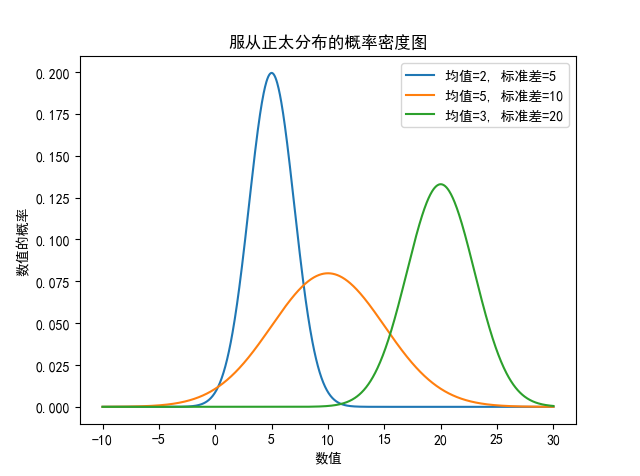展开全文循环输入 正太分布 • 二维高斯分布概率密度函数数据集实战优化坐标轴与图像优化图像再次优化 概率密度函数 大家肯定都有听说过正态分布，其实正态分布只是概率密度分布的一种，正态分布的概率密度函数均值为μ ，标准差σ是高斯函数的一...  二维高斯分布 概率密度函数数据集实战优化坐标轴与图像优化图像再次优化 概率密度函数 大家肯定都有听说过正态分布，其实正态分布只是概率密度分布的一种，正态分布的概率密度函数均值为μ ，标准差σ是高斯函数的一个实例： f ( x ; μ , σ ) = 1 σ 2 π exp ⁡ ( − ( x − μ ) 2 2 σ 2 ) f(x ; \mu, \sigma)=\frac{1}{\sigma \sqrt{2 \pi}} \exp \left(-\frac{(x-\mu)^{2}}{2 \sigma^{2}}\right) 在一维上只有x一个变量，μ 均值，σ标准差。 正态分布具有两个参数μ和σ的连续型随机变量的分布，第一 参数μ是服从正态分布的随机变量的均值，第二个参数σ^2是此随机变量的方差，所以正态分布记作N（μ,σ2）。 实际工作中，正态曲线下横轴上一定区间的面积反映该区间的例数占总例数的百分比，或变量值落在该区间的概率。 因此一维的概率密度分布即正态分布，很好的表示数据在哪个区间集中，使我们对整体数据有一个大概的把握。 本文的重点在于二维概率密度函数： f ( x , y ) = ( 2 π σ 1 σ 2 1 − ρ 2 ) − 1 exp ⁡ [ − 1 2 ( 1 − ρ 2 ) ( ( x − μ 1 ) 2 σ 1 2 − 2 ρ ( x − μ 1 ) ( y − μ 2 ) σ 1 σ 2 + ( y − μ 2 ) 2 σ 2 2 ) ] f(x, y)=\left(2 \pi \sigma_{1} \sigma_{2} \sqrt{1-\rho^{2}}\right)^{-1} \exp \left[-\frac{1}{2\left(1-\rho^{2}\right)}\left(\frac{\left(x-\mu_{1}\right)^{2}}{\sigma_{1}^{2}}-\frac{2 \rho\left(x-\mu_{1}\right)\left(y-\mu_{2}\right)}{\sigma_{1} \sigma_{2}}+\frac{\left(y-\mu_{2}\right)^{2}}{\sigma_{2}^{2}}\right)\right] 因为生活中的很多数据都是高维度的，从简单的二维说起。二维上的数据生活中有很多：身高和体重，血压和血脂等等。如果能够像一维正态分布那样做出图像来看，就十分直观，而本文就是介绍如何作二维概率密度函数的图像。 数据集 首先贴上数据集： 链接：https://pan.baidu.com/s/1RJCwi4-8_hByY6-rCepJgQ 提取码：88ew 数据是截至4.25日的重点国家新冠肺炎感染人数，有中国、美国、法国、意大利等。 本文采取的是中国和意大利进行对比分析。 import numpy as np import matplotlib.pyplot as plt import math import mpl_toolkits.mplot3d import math import pandas as pd data = pd.read_csv('D:/桌面/1.csv') print(data.head()) x = data.iloc[:,1] y = data.iloc[:,7] x = x.values y = y.values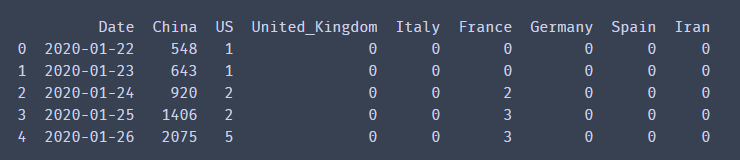实战 首先根据公式我们先把2个维度的均值和方差分别计算出来，以及公式中需要的相关系数。  u1 = x.mean() u2 = y.mean() o1 = x.std() o2 = y.std() from scipy.stats import pearsonr p = pearsonr(x, y) print(u1, u2, o1, o2, p) # 输出:(r, p) # r:相关系数[-1，1]之间 # p:相关系数显著性  相关系数也就是皮尔逊系数，把2个维度数据给入后，会输出相关系数和相关系数显著性。 相关系数取值范围是(-1，1)，越接近1则说明越相关。不过我们也不能说中国感染人数和西班牙感染人数相关，这里更确切地解释应该是感染人数的趋势比较。 X, Y = np.meshgrid(x, y) z = (1/(2*math.pi*o1*o2*pow(1-pow(p,2),0.5)))*np.exp(-1/(2*(1-p*p))*(((X-u1)*(X-u1))/(o1*o1)-2*p*(X-u1)*(Y-u2)/(o1*o2)+(Y-u2)*(Y-u2)/(o2*o2)))  这里X,Y是对原始数据进行网格化，其实就相当于最后成果图的横纵坐标，只是转换一下得以输入作图。 z就是上文的二维密度函数用python来表达了。比较麻烦，注意里面有上面算出的2个维度的均值，方差和皮尔逊系数。 plt.rcParams['font.sans-serif'] = ['KaiTi'] # 用来正常显示中文字符 plt.rcParams['axes.unicode_minus'] = False plt.figure(figsize=(10,10), dpi=300) ax = plt.subplot(111, projection='3d') ax.plot_surface(X, Y, z, cmap='rainbow', alpha=0.9) ax.set_xlabel('中国感染人数') ax.set_ylabel('西班牙感染人数') ax.set_zlabel('频率') ax.set_title("二维高斯分布") plt.savefig('D:/桌面/1.png', bbox_inches='tight', pad_inches=0.0)  这就是很基础的一些画图设置了，相似的就不再赘述，重点 谈谈plot_surface。 plot_surface中的X，Y，z其实上文以及解释过了，就是相应的坐标和函数，那么cmap是什么呢，camp是颜色盘，值定位rainbow就是彩虹色，从下图就可以看出，数据越集中的地方，颜色就越深。这里还有一个颜色盘是coolwarm，不过个人感觉没rainbow好看，不妨小伙伴们试一试。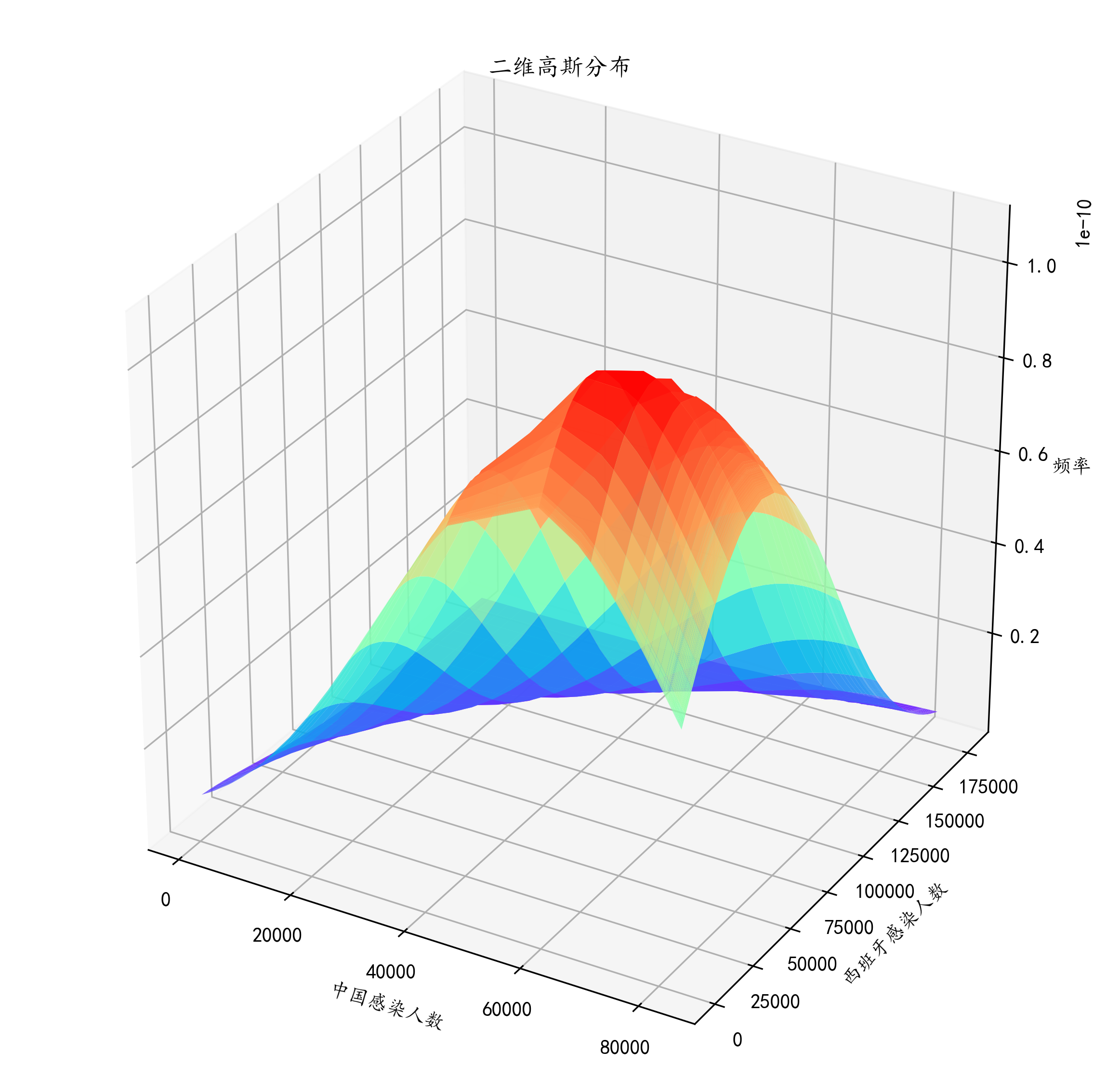到此我们就大概的画出了中国感染人数和西班牙感染人数在4.25之前的密度函数。 这个图我们看出，中国感染人数大概在4-5万就开始达到高峰，之后开始下降，而西班牙到了12万左右才开始下降。整个国家感染人数的增幅一目了然，对于整体数据的把握也有较好的认知。但这样似乎不太好看，而且到底高峰是不是在我说的那个数值呢，根据肉眼都不好判断。所以我们接下来进行优化。 优化 坐标轴与图像优化 plt.rcParams['font.sans-serif'] = ['KaiTi'] # 用来正常显示中文字符 plt.rcParams['axes.unicode_minus'] = False plt.figure(figsize=(10,10), dpi=300) ax = plt.subplot(111, projection='3d') ax.plot_surface(X, Y, z, rstride=1, cstride=1, cmap='rainbow', alpha=0.9) ax.set_xlabel('中国感染人数', fontsize=15) ax.set_ylabel('西班牙感染人数', fontsize=15) ax.set_zlabel('频率', fontsize=15) ax.set_title("二维高斯分布", fontsize=25, y=1.02) ax.set_xticks(np.arange(0,100000,20000)) ax.set_yticks(np.arange(0,200000,40000)) plt.savefig('D:/桌面/3.png', bbox_inches='tight', pad_inches=0.0)  可能一眼还没看出来。我来讲解一下。博主在plot_surface里面加了 rstride=1, cstride=1,这两个参数有什么作用？相当于步长。这么理解吧，这个颜色实际上是由无数个点组成的，但是实际上就像房子顶上的瓦片一样，如果瓦片比较大，那么房顶面积一定，瓦片就用的少，就像上图一样显得一块一块的，非常大，不过不平滑。而下图呢，加入 rstride=1, cstride=1就相当于定制了瓦片长宽，瓦片比较小那么看起来就舒服，颜色过渡得比上面那个自然多。 把标题和坐标轴都修改一下，title的x，y参数是调位置的，如何使用的话小伙伴们多试几个值就明白了。 plt.savefig里面的bbox_inches=‘tight’, pad_inches=0.0在这里看起里效果似乎不明显。这个作用是减小图片旁边的白色区域。如果感兴趣的小伙伴可以试一下不加和加了这些参数保存出来是什么样的。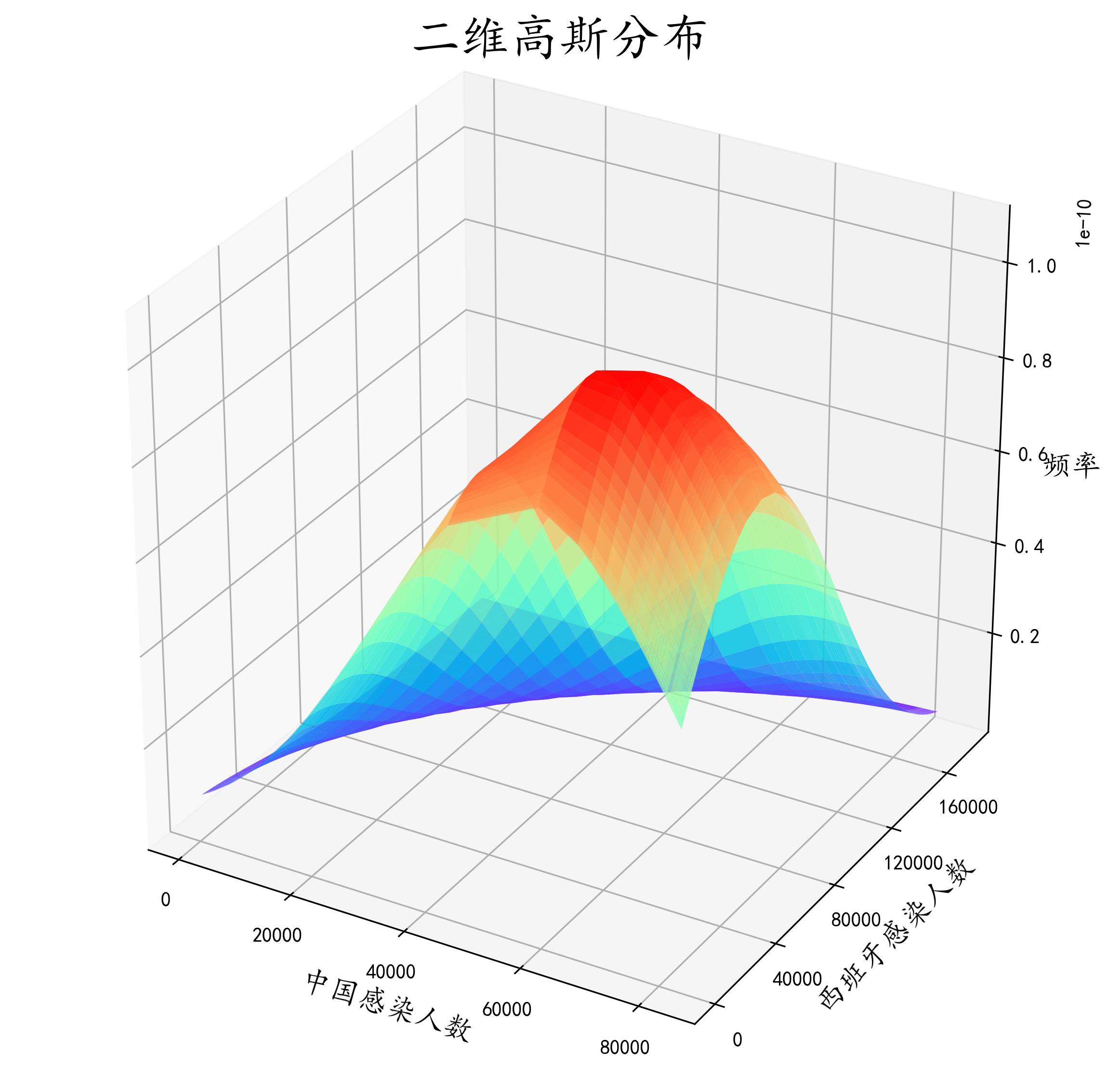图像再次优化 plt.rcParams['font.sans-serif'] = ['KaiTi'] # 用来正常显示中文字符 plt.rcParams['axes.unicode_minus'] = False plt.figure(figsize=(10,10), dpi=300) ax = plt.subplot(111, projection='3d') ax.plot_surface(X, Y, z, rstride=1, cstride=1, cmap='rainbow', alpha=0.9) ax.set_xlabel('中国感染人数', fontsize=15) ax.set_ylabel('西班牙感染人数', fontsize=15) ax.set_zlabel('频率', fontsize=15) ax.set_title("二维高斯分布", fontsize=25, y=1.02) ax.set_xticks(np.arange(0,100000,20000)) ax.set_yticks(np.arange(0,200000,40000)) ax.contour(X, Y, z, 15, zdir = 'z', offset = 0, cmap = plt.get_cmap('rainbow')) ax.w_xaxis.set_pane_color((135/255, 206/255, 250/255, 0.3)) ax.w_yaxis.set_pane_color((135/255, 206/255, 250/255, 0.3)) ax.w_zaxis.set_pane_color((135/255, 206/255, 250/255, 0.3)) plt.savefig('D:/桌面/4.png', bbox_inches='tight', pad_inches=0.0)  作图一方面为了好看，一方面是对数据整体把握更加直观，这里加了ax.w_xaxis.set_pane_color这个方法是对x平面进行上色，个人感觉更好看吧。里面的参数是rgba。 细心的小伙伴已经发现了，这个图比上面的多了好多等高线。这些等高线是这个密度函数在xoy平面的投影，能够更直观的看出到底数据的高峰是在哪。我们直观看出，中国感染人数到达高峰是在6w人左右，而西班牙也是在6w人左右，这和我们前面目测估计的有一点误差。所以ax.contour这个方法将密度函数投影到平面来，更细致的观察数据的分布。其中ax.contour中 15代表是有多少条等高线，zdir=z表示投影到z=？这个平面，而？的数值就是由offset表示，这里显然投影到z=0平面，camp也是和上文意思差不多是颜色盘，彩虹色的。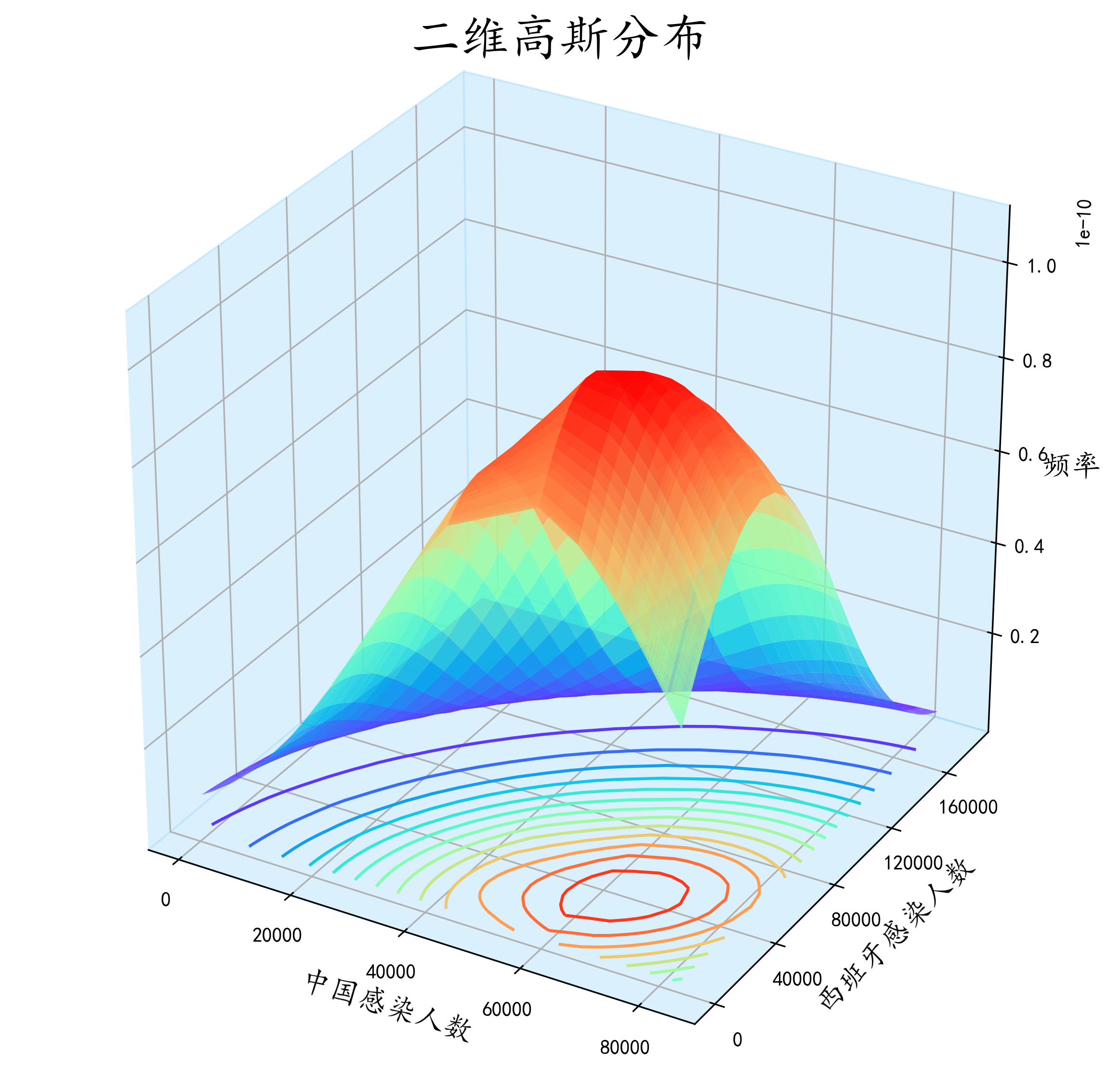效果还是很直观的。 展开全文数据分析 机器学习 • ## 使用Python估计数据概率分布函数 万次阅读 多人点赞 2016-11-23 16:10:47 首先其文档对我这种六级低空飘过的人来说读着好烦恼，所以，对其参数设置什么的不是很理解，从而导致得到的pdf(概率密度函数)效果不是很好。 Plan B 继续使用Seaborn模块 后来我转念一想，seaborn返回的图中...数据 • beta分布的最大特点是其多样性, 从下图可以看出, beta分布具有各种形态, 有U形, 类似正态分布的形状, 类似uniform分布的形状等, 正式这一特质使beta分布在共轭先验的计算中起到重要作用: import matplotlib.pyplot ... • 鉴于单峰的状态分布公式是： f(x)=(1/((2π)^0.5)*σ)exp-((x-a)^2/(2*σ^2)) 于是N个平均值。...这里每个i对应一个独立的平均值ai、标准差σi,以及它的概率峰位置，而各个ki是一个系数，并且它们... • 计算分布函数(也称累积概率函数CDF) python代码如下： import numpy as np data = np.random.randn(200) # 随机生成一些数 # 一个公式即可计算出概率函数 cdf_value = 1. * np.arange(len(data)) / (len(data) - 1... • ## python画概率分布图 千次阅读 2019-10-29 17:49:08 import seaborn as sns sns.kedplot(df, shade = True) • python实现描述性统计、频数分布图、正态分布检验、概率密度曲线拟合 from scipy.stats import chi2 # 卡方分布 from scipy.stats import norm # 正态分布 from scipy.stats import t # t分布 from scip...数据分析 正态分布检验 • ## python plot hist 密度图 概率和不为1 千次阅读 多人点赞 2018-11-23 15:43:33 使用ax.hist()函数想要把数据转为密度直方图，但发现直接使用density=true得到的值很奇怪，y轴甚至会大于1，不符合我的预期。 查了资料发现density=ture的意思是保证该面积的积分为1，并不是概率和为1，因此我们需要... • 一、实现结果： 二、源码 import numpy as np from scipy.stats import beta, norm, uniform, binom import matplotlib.pyplot as plt from functools import wraps def my_plot(label0=None, ... • 直方图的时候，可以叠加个核密度函数，图也很好看，如果对当面这一列数据，画图之后，想知道累积概率那如何获得呢，也就是cdf，pdf倒是容易获得，很多函数直接就有，因为用的多，网上一搜也都能搜到。但是获取累积...scipy • 概率密度函数 概率密度函数（PDF）。 随机变量的（PDF）为 其中mu是平均值，而sigma > 0是标准偏差。 安装$ npm install distributions-normal-pdf 要在浏览器中使用，请使用 。 用法 var pdf = require ( '...JavaScript
• miu = 0 sigma = 1 x = np.linspace(miu - 3 * sigma, miu + 3 * sigma, 50) y = np.exp(-(x - miu) ** 2 / (2 * sigma ** 2)...plt.title('高斯分布函数', fontsize=18) plt.grid(True) plt.show()标准正态分布 matplotlib
• 推荐阅读：如何理解概率分布函数和概率密度函数 文章目录 正态分布(Nomal Distribution) 两点分布 /伯努利分布(Two Point Distribution) 二项分布 (Binomial Distribution) 几何分布 (Geometric Distribution) 泊松...统计学
• 这篇文章通俗地解释了概率论的两个基石函数：概率分布函数、概率密度函数，建议不熟悉的同学，认真阅读。1先从离散型随机变量和连续性随机变量说起对于如何分辨离散型随机变量和...
• 这里我们使用概率密度函数，来解决这个问题，hr给了个限定标准，作为她可以接受的一个计算结果的预期值： 如果计算的结果的概率，在总体点估计量：概率P的[-0.65, +0.65]区间内，就可以接受这个求得的结...数据挖掘
• from scipy import stats # F分布 stats.f.ppf(0.95,n1,n2) # 0.95分位数求概率面积 stats.f.cdf(5.14,n1,n2) # 面积求概率----累计分布 stats.f.pdf(2,n1,n2) # 求x=2的概率----概率分布函数
• 简单对概率分布函数进行一个描述，在概率论中要研究一个随机变量ξ取值小于某一数值x的概率，这概率是x的函数，称这种函数为随机变量ξ的分布函数。 F(x)=P(X<x),F(+∞)=1,F(−∞)=0F(x)=P(X<x),F(+\infin)=1,...
• 我这里并不是要讲“伪随机”、“真随机”这样的问题，而是关于如何生成服从某个概率分布的随机数（或者说 sample）的问题。比如，你想要从一个服从正态分布的随机变量得到 100 个样本，那么肯定抽到接近其均值的样本...正态分布 随机数...

# python画概率密度函数python 订阅